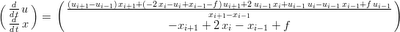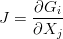## Thursday, June 18, 2009

### Implicit-Time Burgers' Equation on a Moving Grid

In the last post on solving Burgers' equation on a moving grid we ended up with the semi-discrete equation for the rates:where the spatial derivatives (for the solution and the grid) are approximated by simple central differences. We still haven't committed to a time-integration scheme. This is one of the nice things about the method of lines, we can play with different time integration schemes independent of our spatial discretization.

A good way to start out with implicit integration schemes is with the basic Backward Euler formula:where the big X represents the unknown vector and the F(X) represents the right-hand side of our semi-discrete equation. The superscripts represent the time level. The reason this is an implicit time integration method is that the spatial terms are evaluated at the next time level rather than the present time level. This substitution is easily made in Maxima (just like the spatial derivatives):

/* substitute in a backward time difference: */
Discrete_Rate : ratsubst((u[i] - u[i,n-1])/dt, 'diff(u,t), Rate_Vector);
Discrete_Rate : ratsubst((x[i] - x[i,n-1])/dt, 'diff(x,t), Discrete_Rate);

Once the choice of time integration scheme is made, we have a fully-discrete problem that we can solve.The Newton expression, G, in Maxima:

/* expression for Newton's method (zero finding): */
Newton_expression : fullratsimp(Discrete_Rate - Rate_expression);

Since G is non-linear, we'll need to use a Newton's method to solve for the zero (which will give us the solution and grid point locations at the next time-step). This requires the Jacobian:/* Jacobian of the Newton expression: */
J : diff(Newton_expression, u[i-1]);

The update for each iteration of the Newton's method is given by:Of course we generally don't invert the Jacobian directly to find the update vector, usually an iterative method such as Successive Over Relaxation or a Krylov subspace method is preferred. Most of these methods require a function which returns the application of the Jacobian on the update vector, so we go ahead and find that in Maxima as well:

/* Jacobian applied on the update vector: */
update_vector : transpose([du[i-1], du[i], du[i+1], dx[i-1], dx[i], dx[i+1]]);
Jdu : factor(J.update_vector);

The great thing about defining the numerical scheme in Maxima this way is that the f90() function will output these (increasingly complex) expressions to Fortran with the proper array indexing. If you've done this upfront part properly they are ready to go into loops as is.